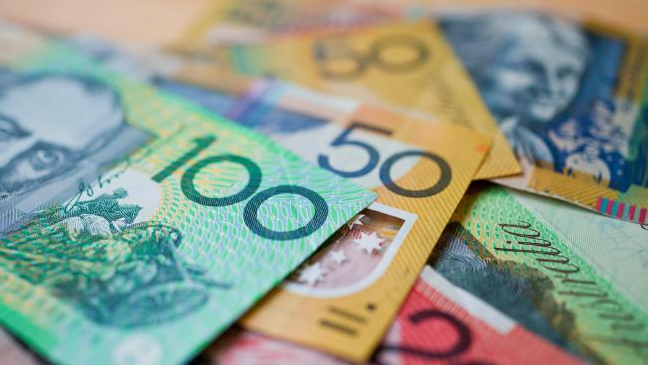# Definition of ProfitA profit is the money you make after all your expenses are paid.

For example, if Sam buys a bicycle for $\150$ and then sells it the next day for $\200$, then his profit is $\200 - \150 = \50$.

If Sam spends another $\25$ on fixing the same bicycle up before he sells it for $\200$, then his profit is $\200 - \150 - \25 = \25$.

If you end up with income that is lower than your expenses, you make a loss.

For example, if Sam buys a bicycle for $125$, spends $\30$ on repairing it, and then sells it for $150$, he makes a loss of $\5$.

### Description

The aim of this dictionary is to provide definitions to common mathematical terms. Students learn a new math skill every week at school, sometimes just before they start a new skill, if they want to look at what a specific term means, this is where this dictionary will become handy and a go-to guide for a student.

### Audience

Year 1 to Year 12 students

### Learning Objectives

Learn common math terms starting with letter P

Author: Subject Coach
You must be logged in as Student to ask a Question.